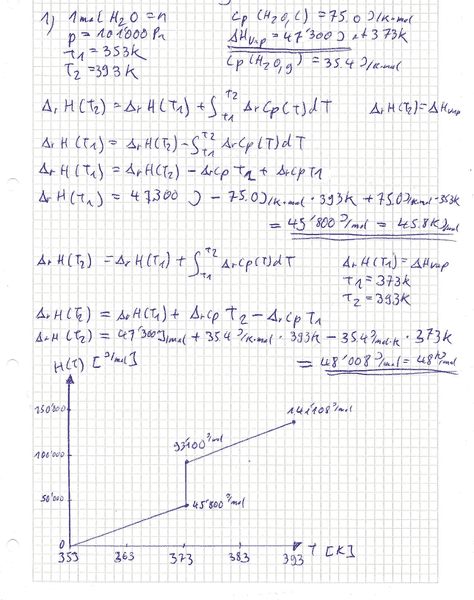# Enthalpy ΔH of H2O

## Homework Statement

What is the enthalpy ΔH, when 1 mole of water is heated at 101 kPa from 353 K to 393 K? Outline the process in an H (T) diagram.
The following values are available:
Cp (H2O, l) = 75.0 J K-1 mol-1; ΔHvap = 47.3 kJ mol-1 at 373 K; Cp (H2O, g) = 35.4 J K-1 mol-1

## The Attempt at a Solution

I tried to solve this:Is this the right way?

Chestermiller
Mentor
I was unable to read what you wrote. The diagram looks OK qualitatively, but not quantitatively. The changes from 353 to 373 for the liquid and from 373 to 393 for the vapor are way too large. The first change is only 1500 J, and the second change is only 708 J. The enthalpy change for vaporization is shown correctly.

Chet

My writing is not the best, sorry

Ok, therefore I calculated it wrong.

For the first ΔH, do I have to calculate ΔH(373K)=ΔH(353K)+(Cp (H2O, l))*373K-(Cp (H2O, l))*353K?

And for the second ΔH, ΔH(393K)=ΔH(373K)+(Cp (H2O, g))*393K-(Cp (H2O, g))*373K?

Chestermiller
Mentor
My writing is not the best, sorry

Ok, therefore I calculated it wrong.

For the first ΔH, do I have to calculate ΔH(373K)=ΔH(353K)+(Cp (H2O, l))*373K-(Cp (H2O, l))*353K?

And for the second ΔH, ΔH(393K)=ΔH(373K)+(Cp (H2O, g))*393K-(Cp (H2O, g))*373K?
It goes like this:

H(373,l)=H(353,l)+Cp(H2O,l)*(373-353)

H(373,v)=H(373,l)+ΔHv(373)

H(393,v)=H(373,v)+Cp(H2O,v)*(393-373)

Chet

Ok, now I'm a bit confused, because you don't use ΔH but H. And also which stage is which in the diagram?

So I start at 353K. Then the first ΔH is from the beginning to the point, where the temperature reaches 373K. This means until the point H(373,l)?

And then the water gets vaporized, which is ΔHv(373)?

And at the end, it goes from the end of ΔHv(373) to 393K, which means the ΔH goes from the end point of ΔHv(373) to H(393,v)?

Chestermiller
Mentor
Ok, now I'm a bit confused, because you don't use ΔH but H. And also which stage is which in the diagram?

So I start at 353K. Then the first ΔH is from the beginning to the point, where the temperature reaches 373K. This means until the point H(373,l)?

And then the water gets vaporized, which is ΔHv(373)?

And at the end, it goes from the end of ΔHv(373) to 393K, which means the ΔH goes from the end point of ΔHv(373) to H(393,v)?
Yes. This is all correct. So, the ΔH you are looking for is ΔH=H(393,v) - H(373,l). This is the change in enthalpy between the initial state and the final state.

•krootox217
But in the diagram, I need to draw in the three ΔH's like i did in the first attempt?

Chestermiller
Mentor
But in the diagram, I need to draw in the three ΔH's like i did in the first attempt?
If that's what they're asking for. In your diagram, you took as a basis H(353,l) = 0 (which is perfectly acceptable).

•krootox217